Courses

# Electric Capacitance Energy Of An Electric Field (Part - 1) - Electrodynamics, Irodov JEE Notes | EduRev

## JEE : Electric Capacitance Energy Of An Electric Field (Part - 1) - Electrodynamics, Irodov JEE Notes | EduRev

The document Electric Capacitance Energy Of An Electric Field (Part - 1) - Electrodynamics, Irodov JEE Notes | EduRev is a part of the JEE Course I. E. Irodov Solutions for Physics Class 11 & Class 12.
All you need of JEE at this link: JEE

Q. 101. Find the capacitance of an isolated ball-shaped conductor of radius R1 surrounded by an adjacent concentric layer of dielectric with permittivity ε and outside radius R2.

Solution. 101. Let us mentally impart a charge q on the conductor, then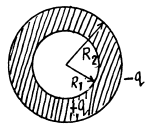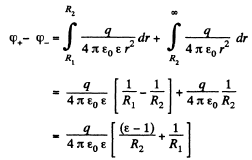Hence the sought capacitance,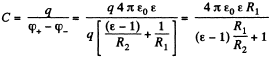Q. 102. Two parallel-plate air capacitors, each of capacitance C, were connected in series to a battery with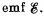Then one of the capacitors was filled up with uniform dielectric with permittivity ε. How many times did the electric field strength in that capacitor decrease? What amount of charge flows through the battery?

Solution. 102. From the symmetry o f the problem, the voltage across each capacitor,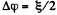and charge on each capacitor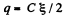in the absence of dielectric.
Now when the dielectric is filled up in one of the capacitors, the equivalent capacitance of the system,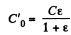and the potential difference across the capacitor, which is filled with dielectric,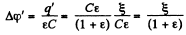But  φα E

So, as φ decreases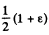times, the field strength also decreases by the same factor and flow of charge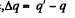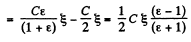Q. 103. The space between the plates of a parallel-plate capacitor is filled consecutively with two dielectric layers 1 and 2 having the thicknesses d1 and d2 and the permittivities ε1 and ε2  respectively. The area of each plate is equal to S. Find:
(a) the capacitance of the capacitor;
(b) the density σ' of the bound charges on the boundary plane if the voltage across the capacitor equals V and the electric field is directed from layer 1 to layer 2.

Solution. 103. (a) As it is series combination of two capacitors,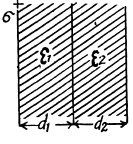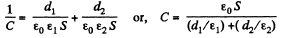(b) Let, a be the initial surface charge density, then density of bound charge on the boundary plane.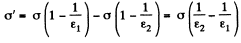But,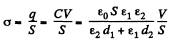So,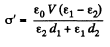Q. 104. The gap between the plates of a parallel-plate capacitor is filled with isotropic dielectric whose permittivity ε varies linearly from ε1 to ε22 > ε1) in the direction perpendicular to the plates. The area of each plate equals S, the separation between the plates is equal to d. Find:
(a) the capacitance of the capacitor;
(b) the space density of the bound charges as a function of ε if the charge of the capacitor is q and the field E in it is directed toward the growing ε values.

Solution. 104. (a) We point the jt-axis lowards right and place the origin on the left hand side plate. The left plate is assumed to be positively charged.

Since e varies linearly, we can write,

ε(x) = a + bx

where a and b can be determined from the boundary condition. We have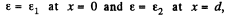Thus,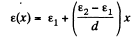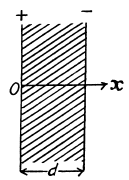Now potential difference between the plates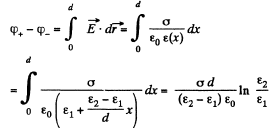Hence, the sought capacitance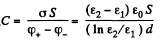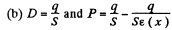and the space density of bound charges is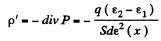Q. 105. Find the capacitance of a spherical capacitor whose electrodes have radii R1 and R2 > R1 and which is filled with isotropic dielectric whose permittivity varies as ε = a/r, where a is a constant, and r is the distance from the centre of the capacitor.

Solution. 105. Let, us mentally impart a charge q to the conductor. Now potential difference between the plates,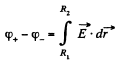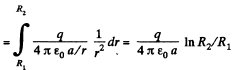Hence, the sought capacitance,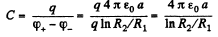Q. 106. A cylindrical capacitor is filled with two cylindrical layers of dielectric with permittivities ε1 and ε2. The inside radii of the layers are equal to R1 and R2 > R1. The maximum permissible values of electric field strength are equal to E1m and E2m  for these dielectrics. At what relationship between ε, R, and Em, will the voltage increase result in the field strength reaching the breakdown value for both dielectrics simultaneously?

Solution. 106. Let λ be the linear chaige density then,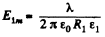(1)
and,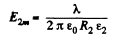(2)

The breakdown in either case will occur at the smaller value of r for a simultaneous breakdown of both dielectrics.

From (1) and (2)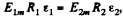which & the sought relationship.

Q. 107. There is a double-layer cylindrical capacitor whose parameters are shown in Fig. 3.16. The breakdown field strength values for these dielectrics are equal to E1 and E2 respectively. What is the breakdown voltage of this capacitor if ε1R1E1< ε2R2E2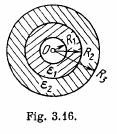Solution. 107. Let, λ be the linear chaige density then, the sought potential difference,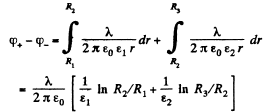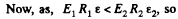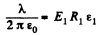is the maximum acceptable value, and for values greater than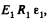dielectric breakdown will take place,

Hence, the maximum potential difference between the plates,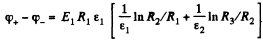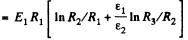Q. 108. Two long straight wires with equal cross-sectional radii a are located parallel to each other in air. The distance between their axes equals b. Find the mutual capacitance of the wires per unit length under the condition b ≫ a.

Solution. 108. Let us suppose that linear chaige density of the wires be λ then, the potential difference,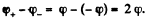The intensity of the electric field created by one of the wires at a distance x from its axis can be easily found with the help of the Gauss’s theorem,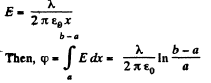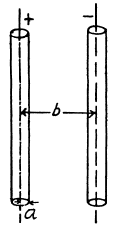Henee, capacitance, per unit length,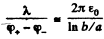Q. 109. A long straight wire is located parallel to an infinite conducting plate. The wire cross-sectional radius is equal to a, the distance between the axis of the wire and the plane equals b. Find the mutual capacitance of this system per unit length of the wire under the condition a ≪ b.

Solution. 109. The field in the region between the conducting plane and the wire can bt obtained by using an oppositely charged wire as an image on the other side.
Then the potential difference between the wire and the plane,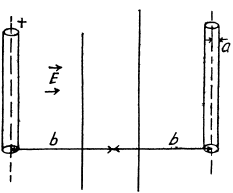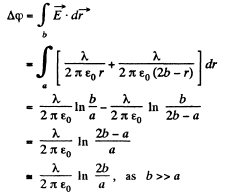Hence, the sought mutual capacitance of the system per unit length of the wire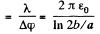Q. 110. Find the capacitance of a system of two identical metal balls of radius a if the distance between their centres is equal to b, with b ≫ a. The system is located in a uniform dielectric with permittivity a.

Solution. 110. When b >> a, the charge distribution on each spherical conductor is practically unaffected by the presence of the other conductor. Then, the potential φ+-) on the positive (respectively negative) charged conductor is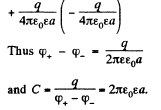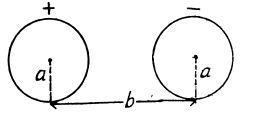Note : if we require tetms which depend on a/b, we have to take account of distribution of charge on the conductors.

Q. 111. Determine the capacitance of a system consisting of a metal ball of radius a and an infinite conducting plane separated from the centre of the ball by the distance l if l ≫ a.

Solution. 111.  As in Q.109 we apply the method of image. Then the potentical difference between the +vely charged sphere and the conducting plane is one half the nominal potential difference between the sphere and its image and is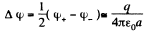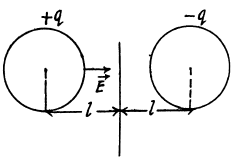Thus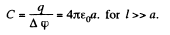Q. 112. Find the capacitance of a system of identical capacitors between points A and B shown in (a) Fig. 3.17a; (b) Fig. 3.17b.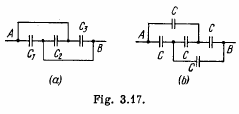Solution. 112.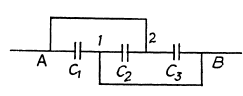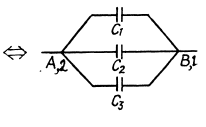(a)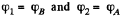The arrangement of capacitors shown in the problem is equivalent to the arrangement shown in the Fig.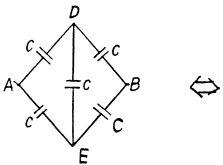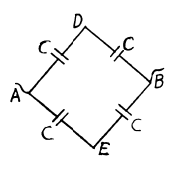and hence the capacitance between A and B is,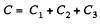(B) From the symmetry of the problem, there is no P.d. between D and E.. So, the combination reduces to a simple arrangement shown in the Fig and hence the net capacitance,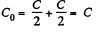Q. 113. Four identical metal plates are located in air at equal distances d from one another. The area of each plate is equal to S. Find the capacitance of the system between points A and B if the plates are interconnected as shown (a) in Fig. 3.18a; (b) in Fig. 3.18b.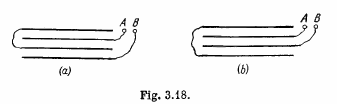Solution. 113. (a) In the given arrangement, we have three capacitors of equal capacitance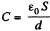and the first and third plates are at the same potential.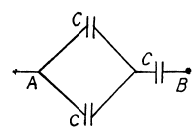Hence, we can resolve the network into a simple form using series and parallel grouping of capacitors, as shown in the figure. Thus the equivalent capacitance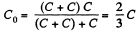(b) Let us mentally impart the charges +q and -q to the plates 1 and 2 and then distribute them to other plates using charge conservation and electric induction. (Fig.). As the potential difference between the plates 1 and 2 is zero,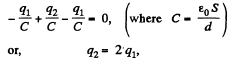The potential difference between A and B,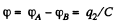Hence the sought capacitane,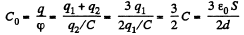Q. 114. A capacitor of capacitance C1 = 1.0 μ.F withstands the maximum voltage V1 =  6.0 kV while a capacitor of capacitance C2 = 2.0 μF, the maximum voltage V2 = 4.0 kV. What voltage will the system of these two capacitors withstand if they are connected in series?

Solution. 114. Amount of charge, that the capacitor of capacitance C1 can withstand, q1 = C1 V1 and similarly the charge, that the capacitor of capacitance C2 can withstand, q2 = C2 V2. But in series combination, charge on both the capacitors will be same, so, qmax, that the combination can withstand = C1V1,

as C1 V1 < C2 V2, from the numerical data, given.

Now, net capacitance of the system,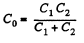and hence,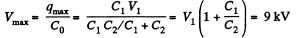Q. 115. Find the potential difference between points A and B of the system shown in Fig. 3.19 if the emf is equal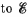= 110 V and the capacitance ratio C2/C1 = η = 2.0.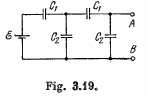Solution. 115. Let us distribute the charges, as shown in the figure.

Now, we know that in a closed circuit, - Δφ = 0

So, in the loop, DCFED,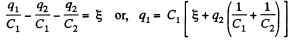(1)

Again in the loop DGHED,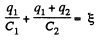(2)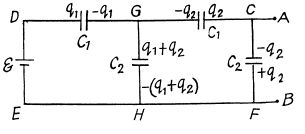Using Eqs. (1) and (2), we get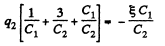Now,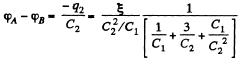or,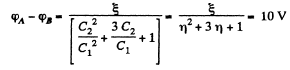Q. 116. Find the capacitance of an infinite circuit formed by the repetition of the same link consisting of two identical capacitors, each with capacitance C (Fig. 3.20).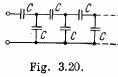Solution. 116. The infinite circuit, may be reduced to the circuit, shown in the Fig. where, C0 is the net capacitance of the combination.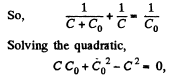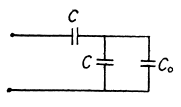we get,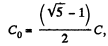taking only +ve value as C0 can not be negative.

Offer running on EduRev: Apply code STAYHOME200 to get INR 200 off on our premium plan EduRev Infinity!

111 docs

,

,

,

,

,

,

,

,

,

,

,

,

,

,

,

,

,

,

,

,

,

,

,

,

;